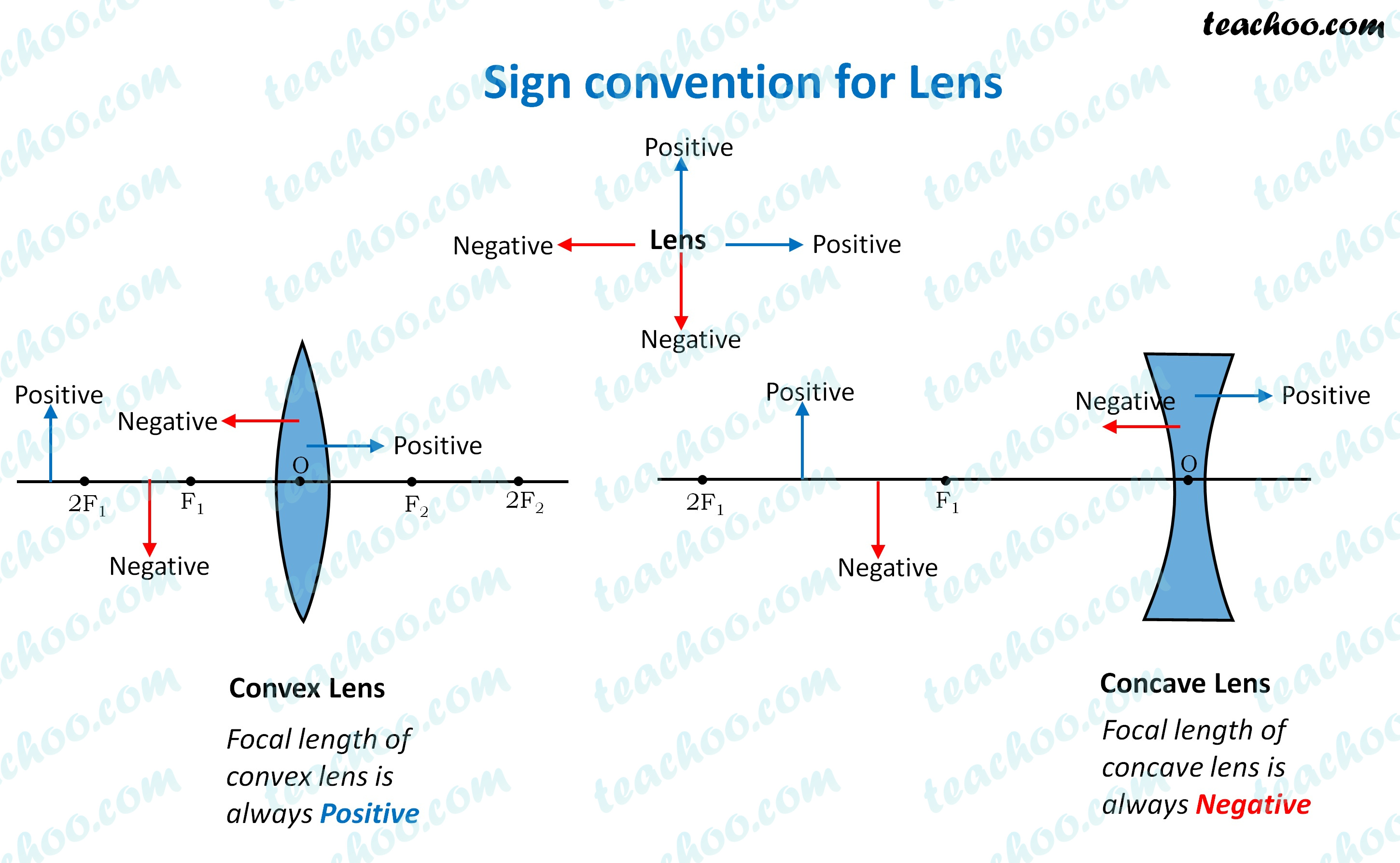NCERT Questions

Class 10
Chapter 10 Class 10 - Light - Reflection and Refraction

## Find the focal length of a lens of power – 2.0 D. What type of lens is this?

Given

Power of lens = –2.0 D

We know that,

Power of the lens = 1/Focal length

–2 = 1/Focal Length

Focal length = 1/(–2)

Focal length = –0.5 m

Since the focal length is negative, the lens is a concave lens.Learn in your speed, with individual attention - Teachoo Maths 1-on-1 Class